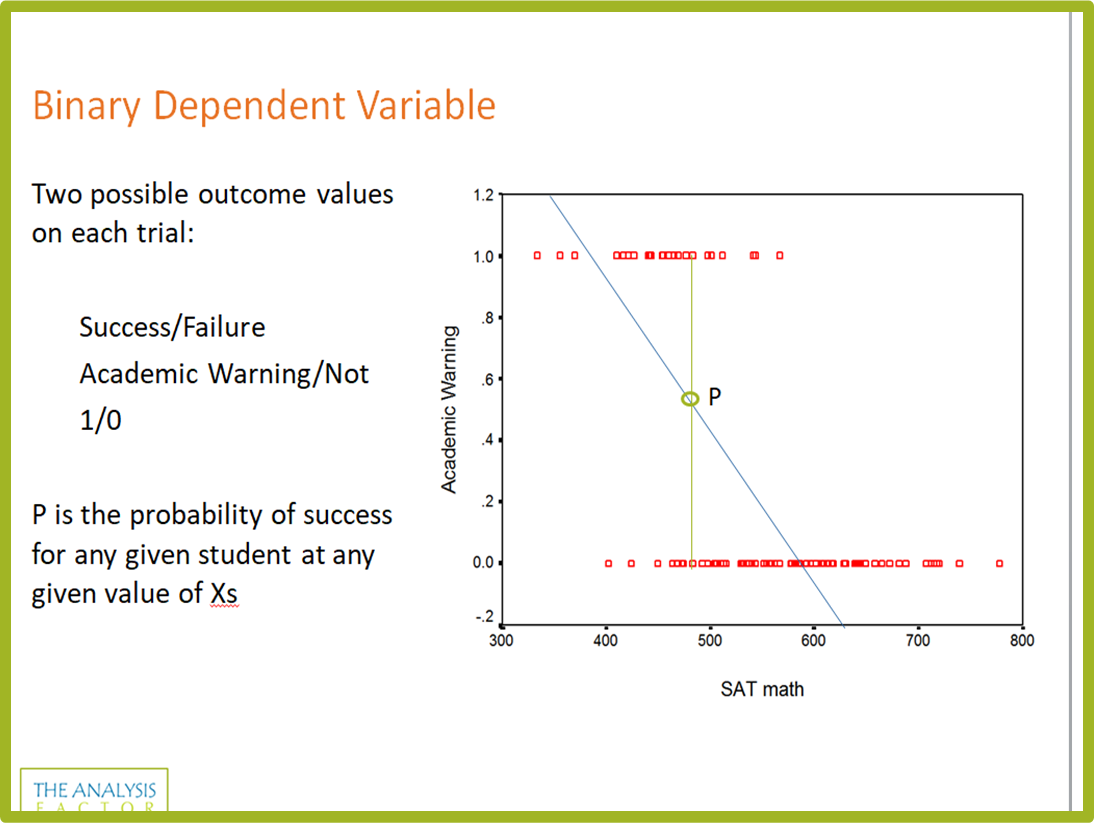Binary, Ordinal, and Multinomial Logistic Regression for Categorical Outcomes

Get beyond the frustration of learning odds ratios, logit link functions, and proportional odds assumptions on your own!
Instructor: Karen Grace-MartinLogistic regression is one of the most useful tools you can have in your statistical tool box.

The different types can be used in a common data situation when linear models can't - when the outcome variable is categorical.

They are a little trickier to learn than linear models, but once you get the idea, you'll see that they're well within your reach.

Covered in this webinar:

1.
The three flavors of logistic regression: binary, nominal, and ordinal for three types of categorical outcomes
2.
How to decide which one to use
3.
How to interpret results
4.
How all three types relate to linear regression